Next: Results Up: Discreteness and its effect Previous: Resonant interaction in discrete

# Numerical simulation

Numerical simulations presented in this work were performed on a single-processor workstation (2.5GHz, 1Gb RAM). We performed a direct numerical simulation, integrating the dynamical equations of motion (4) and (5) using pseudo-spectral method with resolution of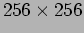wavenumbers. Numerical integrator used for advancing in time was RK7(8) presented in . Time step was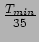where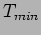is the period of the shortest wave on the axis. Approximate processor time for this work was 4.5 weeks.

In our numerical experiment, we force the system in the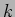-space ring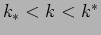with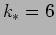and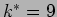. This ring is located at the low wavenumber part of the-space in order to generate energy cascade toward large's, but we deliberately avoid forcing even longer waves (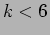) because our experience shows that this would lead to undesirable strong anisotropic effects. In the ring, we fix the shape to coincide with the ZF spectrum,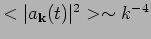, and hence we set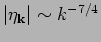,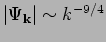. These fixed amplitudes were then multiplied by random phase factors. Thus, surface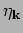and velocity potential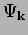were set to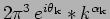, where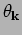were uniformly distributed in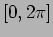and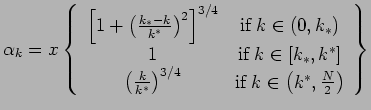where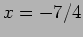for the surface and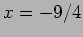for velocity potential. Damping was applied in both small and large wavenumber regions. At small wavenumbers inside the forcing ring, we applied an adaptive damping to prevent formation of undesirable condensate'' which could spoil isotropy and locality of scale interactions. At large wavenumbers, damping is needed to absorb the energy cascade and, therefore, to avoid bottleneck'' spectrum accumulation near the cutoff wavenumber. In our simulations, we implemented the damping as a low-pass filter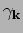applied to the-space variables at each time step. The damping function had the form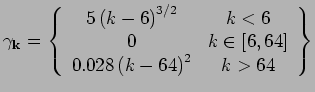Nonlinearity parameter was set to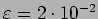, which is a sufficient value to produce a resonance broadening for supporting energy cascade.Next: Results Up: Discreteness and its effect Previous: Resonant interaction in discrete
Dr Yuri V Lvov 2007-01-16